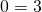# Solving two step equations

These can often be solved by common sense. Two algebraic steps will get there too. Try the ‘common sense’ approach first – ‘what number do you multiply by … then add … to get ….’?

# Solving Linear equal Linear Equation

It can be helpful to follow some predetermined steps to solve these.

• Expand any brackets, simplify each side if necessary;
• Add or subtract theterm from one side so that there is anterm on one side only;
• Continue as for a two step equation.

There could be a better approach than these three steps – it really depends on the equation you’re solving. This is why it is good to keep thinking about the objective (figuring out whatworks) and not get too hung up on one taught method.

On these equations, there is usually just one solution. It is possible however, that there are infinitely many solutions or no solutions at all.

Here is an example of infinitely many solutions:Any value ofwill work for that equation!

Here is an example of no solution:When we work that equation, we get to, which is not true. So no value ofworks for this equation.

# Solving Linear equal Linear Equations with Fractions

Remember that every-now-(but-not-very-often-in-this-applet)-and-again it is possible to have no solution, or to have infinitely many solutions. Answers should be entered as fractions, not as rounded decimals.